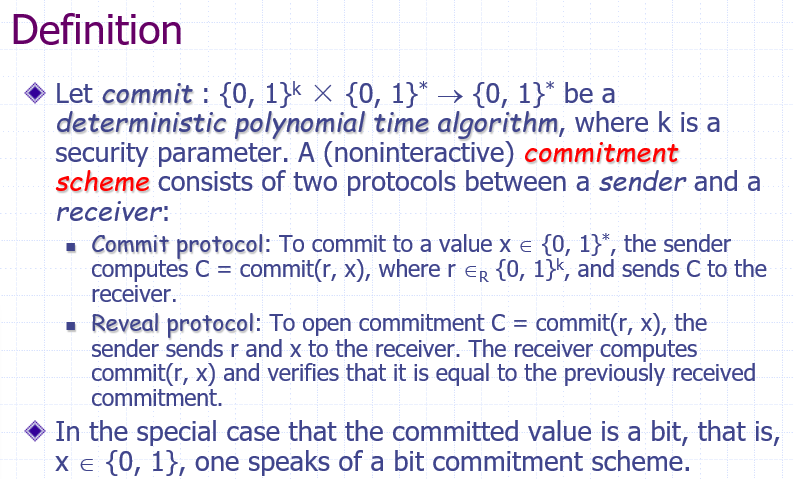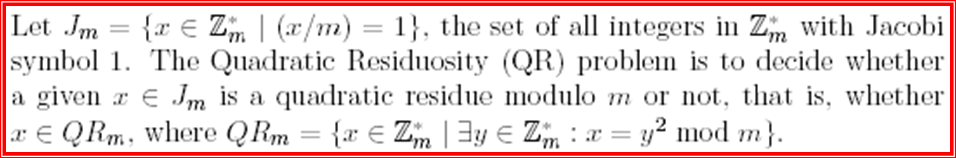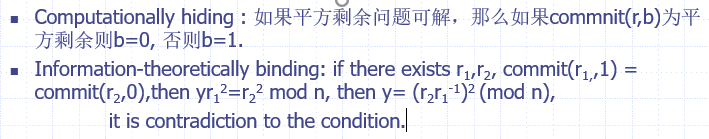《密码协议》课程笔记（2） 比特承诺协议

## 比特承诺协议

### 协议概述

• 可能场景：拍卖、彩票方案、商业谈判、零知识证明，多方协议、盲签名

• 直观含义

• A把承诺的比特放在一个箱子里并锁住，只有A有钥匙，箱子送给B，之后A决定向B证实消息（打开承诺），把钥匙给B，B打开箱子确认A的承诺，且B确信箱子里的消息在保管期间没有被篡改
• A：承诺者，B：验证者
• 承诺阶段：A选择一个要承诺的比特，将能表示比特的信息c发给B
• 打开阶段：A把打开承诺的消息d和b发给B，B用d打开c，验证b是否为承诺的比特
• 安全性质：

• 隐藏性：一个不诚实的接受方无法看到发送方承诺的值
• 绑定性：发送者不能在打开承诺阶段改变自己承诺的比特
• 协议同时满足两个性质才称为安全；如果性质的成立依赖于某个计算困难假设，则称其是计算上绑定的，或计算上隐藏的；如果不依赖，则认为是信息论上绑定的、隐藏的
• 比特承诺方案是由两方（发送方与接收方）参与的、分为两个阶段（承诺阶段与打开阶段）执行的协议，满足隐藏性和绑定性• 可能存在中间人攻击：攻击者在提交承诺时用$$C'$$替换$$C$$，在打开时用$$r',x'$$替换$$r,x$$

• 比特承诺对验证者有些不公平

• 比特承诺不是公开可验证的——和公钥签名不同

• 不存在信息论隐藏和信息论绑定的比特承诺协议

### 常用的比特承诺协议

#### 基于加密

• 基于对称密钥

• 加密算法E，解密算法D，$$||$$表示将两个字符串合并
• 承诺阶段：
1. B产生一个随机比特串r，发给A
2. A选择承诺的比特b，随机产生密钥K，将$$E_K(r||b)$$发给B
• 打开阶段：
1. A发送K给B
2. B解密消息，验证其中的随机串是否和r一致，验证承诺的有效性
• 若要作假，A必须能找到一个新的消息，不仅使承诺的比特反转，还准确产生B的随机串r
• 基于RSA

• $$K=(n=pq,e,d)$$为RSA密钥

• 承诺阶段：

1. A随机选择x，x为奇数则b为0，为偶数则b为1

2. A发送$$x^e\ mod\ n$$给B

• 打开阶段：B使用RSA解密即可

• 隐藏性：b不参与运算；绑定性：不存在奇数的$$x_1$$和偶数的$$x_2$$使得$$x_1^e=x_2^e\ mod\ n$$

• 平方剩余问题

• $$K=(n=pq,m\notin QR(n))$$，公开n和m
• 承诺阶段：选择随机的x，使得$$Y=f(b,x)=m^bx^2\ mod\ n$$
• 打开阶段：发送b和x，B重复加密过程检查结果是否一致
• 隐藏性：若平方剩余问题不可解，则b的信息不会泄露；绑定性：若不绑定，则存在$$x_1,x_2$$使得$$mx_1^2=mx_2^2 \mod n$$，则$$m=(x_2x_1^{-1})^2\ mod\ n$$，矛盾

#### 基于Hash函数

• 承诺阶段：
1. A随机产生两个比特串$$r_1,r_2$$
2. A发送$$H(r_1||r_2||b)$$$$r_1$$给B
• 打开阶段：
1. A将原始消息$$r_1||r_2||b$$发给B
2. B计算散列值，并对比收到的承诺和$$r_1$$
• 隐藏性：哈希的单向性保证；绑定性：哈希的抗碰撞性保证——计算隐藏和计算绑定的

#### 基于离散对数

• Pedersen：
• $$g$$$$Z_p^{*}$$的生成元，令$$h$$为一个随机的群元素，因此$$h$$的阶是保密的
• 承诺阶段：（commit(r,b): b为待commit的信息，r为一个随机值）
1. A选择比特b，从$$Z_p^*$$中选一个随机元素$$r$$
2. 计算$$c=g^rh^b\ mod\ n$$，发送给B
• 打开阶段：
1. 将r和b发给B
2. 验证c的计算结果是否一致
• 隐藏性：由于r是随机选择的，因此B无法区分$$c_0=g^r\ mod\ p$$$$c_1=g^rh\ mod\ p$$，即$$g^r,g^rh$$的分布是统计不可区分的；绑定性：若A要修改，A要重新计算随机数h的离散对数，这是计算困难问题——信息论隐藏、计算绑定
• 信息论隐藏：commit(r,0)commit(r,1)统计不可区分

• ElGamal-like：
• 发送$$g^r$$$$h^{r+b}$$
• 计算隐藏：基于DDH问题——给定结果来求b是DDH困难的
• 信息论绑定——不存在$$r,r'$$使得结果相等，即不能又作为0又作为1打开承诺

#### 同态承诺

• 具有性质“保持两个域上运算不变”的承诺，能够在提交的承诺做计算而不失去承诺的安全性（隐藏、绑定）
• 举例：Pedersen协议是同态的：$$commit_1(r,x)commit(r',x')=commit_1(r+r',x+x')$$
• 右边的乘积计算在群$$G$$中进行，左边的加法计算在$$Z_n$$中进行——两个承诺的乘积，是承诺值的和
• 可以作为安全选举的一部分：投票人将选票放入同态承诺，计数阶段，选票通过取所有承诺的乘积来计数)

• $$commit(r,b)=y^br^2\ mod\ m,r\in Z_m$$• b为0，当且仅当$$commit(r,b)$$为一个m的二次剩余
• 同态性质：$$commit_1(r,x)commit(r',x')=commit_1(rr',x\bigoplus x')$$### 应用

• 抛硬币
• A和B通过抛硬币做决定，二者位于两个不同地方
• A和B各自选择一个随机比特，各自对选择的比特作承诺。最后都打开自己的承诺，抛硬币结果是选择的随机比特的异或
• 拍卖
• 零知识：A向B证明A拥有某种知识，但是证明过程不向B透露知识到底是什么